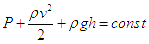# Bernoulli’s Equation

## Incompressible Flow

For incompressible fluids, Bernoulli's equations simply states that the combined kinetic energy, gravitational static energy and pressure head for a flow remains constant. Mathematically this can be stated as,The first term on the left hand side of this equation is clearly the pressure head of the flow at a point. Second term is the kinetic head and the third term is the gravitational static head. As per Bernoulli's equation, for an incompressible flow, the sum of these three remains constant. It should be noted that the frictional loss of energy has been neglected here. That is however never the case for real incompressible flows.

## Compressible Flow

Bernoulli's equation is sometimes valid for the flow compressible flows or gas flows. For Bernoulli's equation to be valid for gases, there should be no transfer of kinetic or potential energy from the gas flow to the compression or expansion of the gas. In other words the compressible gas should behave as an incompressible fluid for Bernoulli's equation to be valid. For a gas to behave as an incompressible fluid, the gas density must remain constant and as per the ideal gas law, this is possible only in case of isobaric (constant pressure) or isochoric (constant volume) processes. Thus the gas flow should be either isobaric or isochoric. In other words pressure and volumetric flowrate can never change simultaneously.